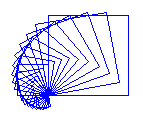deutsch     english    français     Print

## 2.6 VARIABLES### INTRODUCTION

In the previous chapter, you drew squares with side lengths that were firmly implemented in the program. There may also be times when you want to enter the side length with an input dialog. In order to do this, the program needs to store the entered number as a variable. You can see the variable as a container, the content of which you can access with a name. So, in short, a variable has a name and a value. You can freely choose the name of a variable, but they cannot be keywords or names with special characters. Moreover the name cannot start with a number.

 With the notation a = 2 you create the container that you can access with the name a and put the number 2 inside. In the future we will say that you are defining a variable a and assigning a value to it.a = 2 : variable definition (assignment) You can only put one object into the container. Later in your program, when you want to store another number 3 under the name a, write a = 3 [more...In Python are also numbers objects. a is a reference variable and a new assignment creates a new object. The old object is removed by the Garbage Collector.].a = 3 : new assignment

So then what happens when you write a = a + 5? You take the number that is currently in the container, accessed with the name a, and therefore the number 3 is added to the number 5. The result adds up to 8 and it is again saved under the name a.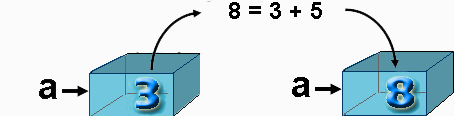Therefore, the equal sign does not mean the same thing in computer programming as it does in mathematics. It does not define an equation, but rather a variable definition or an assignment [more...In some programming languages are used therefore for assigning a different notation, for example, : = or MAKE].

PROGRAMMING CONCEPTS: Variable definition, assignment

### READING AND MODIFYING VARIABLE VALUES

 You can assign a value between 10 and 100 to the variable x with the help of the dialog box. You change this value in the following looping structure, which results in drawing a spiral.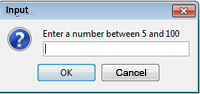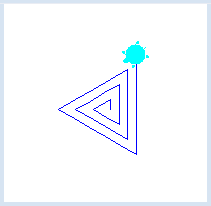```from gturtle import *

makeTurtle()

x = inputInt("Enter a number between 5 and 100")
repeat 10:
forward(x)
left(120)
x = x + 20
```
Highlight program code (Ctrl+C copy, Ctrl+V paste)

### MEMO

 With variables you can store values that you are able to read and change in the course of the program. Every variable has a name and a value. You can define a variable and assign a value to it using an equal sign [more... Values of variables are stored in the computer's memory and will be lost when the program ends or the computer is turned off.].

### DISTINGUISHING VARIABLES AND PARAMETERS

You should be aware of the differences between a variable and a parameter. Parameters transport data into a function and are only valid within that function, whereas variables are possible anywhere. When calling a function, you give each of its parameters values that can be used as variables within the function's scope [more... A parameter is an entrance gate to transfer data into the interior of the function].

 To make the difference clear, use the parameter sidelength in the function square() in your program. When you input a number with inputInt(), it stores it as the variable s. When you call square(), you then pass the variable value of s on to the parameter sidelength.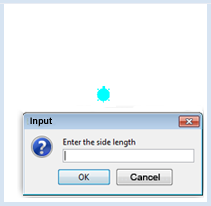```from gturtle import *

def square(sidelength):
repeat 4:
forward(sidelength)
right(90)

makeTurtle()
s = inputInt("Enter the side length")
square(s)
```
Highlight program code (Ctrl+C copy, Ctrl+V paste)

### MEMO

 You have to distinguish between the variable s and the parameter sidelength. In the definition of a function parameters are placeholders and can be regarded as variables that are only known inside of the function each time it is called. If you call the function with a variable, the variable's value is used in the function. Thus, square(length) draws a square with a side length of length [more... The variable value is used as the parameter value (pass-by-value)].

### THE SAME NAME FOR DIFFERENT THINGS

 As you already know, parameters and variables should be named after what they relate to, but they can be chosen arbitrarily. Because of this, it is common to choose the same name for parameters and variables. No naming conflicts arise. However, you must remember the distinction in order to understand the program.```from gturtle import *

def square(sidelength):
repeat 4:
forward(sidelength)
right(90)

makeTurtle()
sidelength= inputInt("Enter the side length")
square(sidelength)
```
Highlight program code (Ctrl+C copy, Ctrl+V paste)

### MEMO

 Although you can use the same name for a specific parameter and variable, you should be able to conceptualize them separately.

### EXERCISES

1.

 After entering the number of corners into the dialog box, the turtle should draw a regular n-gon. For example, when you input the number 8, an 8-gon (octagon) should be drawn. The program should calculate the appropriate rotation angle. Put yourself in the position of the turtle and think how far you have to rotate yourself in order to draw the next side. Remember how we drew an equilateral triangle. .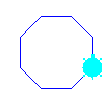2.

 After entering an angle in the dialog box, the turtle draws 30 lines, each with a side length of 100, and after each of which it rotates left by the given angle. Experiment with different angles and draw some cool pictures. You can speed up the drawing by with hideTurtle().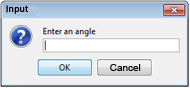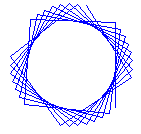3.

 Tell the turtle to draw 10 squares. First define a command square with the parameter sidelength. The side length of the first square is 8, and each following square has a side length increasing by 10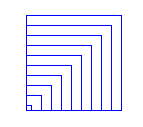4.

 Enter the side length of the largest square into the dialog box. The turtle will then draw 20 squares. After each previous square, the side length should be smaller by a factor of 0.9 and the turtle should rotate 10° to the left